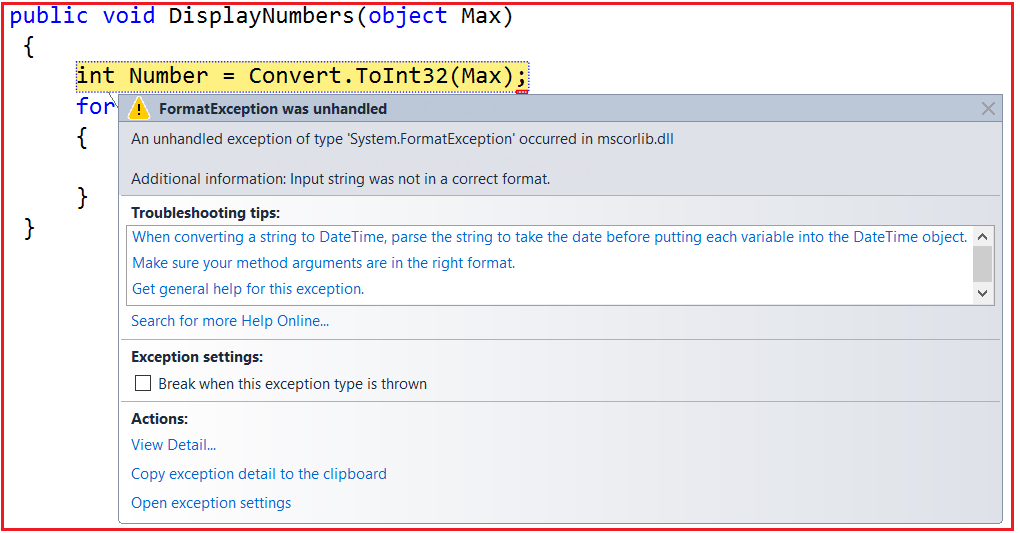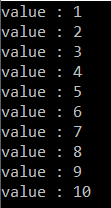# How to Pass Data to Thread Function in Type Safe Manner in C#

## How to Pass Data to Thread Function in Type-Safe Manner in C#

In this article, I am going to discuss How to Pass Data to Thread Function in Type-Safe Manner in C# with Examples. Please read our previous article where we discussed the Thread Class in C# with Examples. As part of this article, we are going to discuss the following pointers.

1. How to Pass data to the Thread function in C#?
2. How to make the thread function type-safe in C#?
##### How to Pass Data to the Thread Function in C#

Let us understand this with an example. In the below example, the DisplayNumbers function takes an argument of the object type. Then in the main method, we created an instance of the ParameterizedThreadStart delegate, and to the constructor of the ParameterizedThreadStart delegate, we pass the DisplayNumbers function. Next, we created an instance of the Thread class and to the constructor of the Thread class, we pass the ParameterizedThreadStart delegate instance as a parameter that points to the DisplayNumbers function. Finally, we call the Start method and pass a string value “Hi”, and here we will not get any compile-time error.

```using System.Threading;
using System;
{
class Program
{
static void Main(string[] args)
{
Program obj = new Program();

t1.Start("Hi");
}

public void DisplayNumbers(object Max)
{
int Number = Convert.ToInt32(Max);
for (int i = 1; i <= Number; i++)
{
Console.WriteLine("Method1 :" + i);
}
}
}
}```

At the time of compilation, we will not get any compile-time error. But when we run the application, we will get the following runtime error.This is because the thread function is not type-safe as it operates on the object data type. Let’s see how to make the thread function type-safe so that we can pass the data in a type-safe manner. So, whenever we are saying type safe means there should be any kind of boxing and unboxing.

##### How to Make the Thread Function Type-Safe in C#?

When we are saying type-safe, it means we should not use the object data type. Here in our example, we need to use the data type as an integer. So at the time of compilation, if we pass any data other than an integer, then it should give us a compile-time error. Let us see how to achieve this step by step.

###### Step1:

In order to pass the data in a type-safe manner to a Thread function in C#, first, you need to encapsulate the thread function and the data it requires in a helper class. So, create a class file with the NumberHelper.cs and then copy and paste the following code into it.

```using System;
{
public class NumberHelper
{
int _Number;

public NumberHelper(int Number)
{
_Number = Number;
}

public void DisplayNumbers()
{
for (int i = 1; i <= _Number; i++)
{
Console.WriteLine("value : " + i);
}
}
}
}```

As you can see, we have created the above NumberHelper class with one private variable i.e. _Number, one parameterized constructor, and one method i.e. DisplayNumbers. The private variable _Number is going to hold the target number. The constructor takes one input parameter of integer type and then assigns that value to the private variable. So, while we are creating the instance of NumberHelper class we need to supply the Number value. Finally, the DisplayNumbers function is used to display the values starting from 1 to the value that is present in the _Number variable.

###### Step2:

In the main method, we need to create an instance of NumberHelper class and to its constructor, we need to pass the integer value. Then create the ThreadStart delegate instance and pass the DisplayNumbers function as a parameter to its constructor. So, please modify the Program.cs class file as shown below.

```using System.Threading;
using System;
{
class Program
{
static void Main(string[] args)
{
int Max = 10;
NumberHelper obj = new NumberHelper(Max);

T1.Start();
}
}
}
```

Now run the application and it should display the output as expected as shown below.In the next article, I am going to discuss How to Retrieve Data From a Thread Function in C# with Examples. Here, in this article, I try to explain How to Pass Data to a Thread Function in a Type-Safe Manner in C# with Examples. I hope you enjoy this How to Pass Data to Thread Function in Type-Safe Manner in C# with Examples article.

### 2 thoughts on “How to Pass Data to Thread Function in Type Safe Manner in C#”

1.Heres a code that use generics:

``` using System; using System.Collections.Generic; using System.Linq; using System.Text; using System.Threading.Tasks; using System.Threading; namespace Destructor { public class Helper { private List data; public Helper(List list) { this.data = list; } public void Display() { foreach (T element in this.data) { if (element is T x) { MainProgramMultiThreading.println(\$"Value: {x}"); } } } } public class MainProgramMultiThreading { public static Action println = new Action(Console.WriteLine); public static Action print = new Action(Console.Write); public static void Main(string[] args) { string[] arr = { "Ignacio", "Elizabeth", "Juan" }; List nombres = new List(arr); Helper objHelper = new Helper(nombres); Thread thread = new Thread(objHelper.Display); thread.Start(); } } } ```

2.public class ActionHelper
{
public ActionHelper(T parameter,Action actionWithParameter)
{
this.parameter = parameter;
action = actionWithParameter;
}

public void Handler()
{
action.Invoke(parameter);
}
}
ActionHelper actionHelper = new ActionHelper(“Y79H9h@9%1”, Display);
t1.Start();